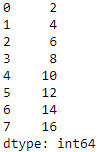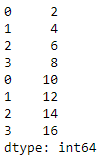# Add a Pandas series to another Pandas series

Let us see how to add a Pandas series to another series in Python. This can be done using 2 ways:

Method 1: Using the append() function: It appends one series object at the end of another series object and returns an appended series. The attribute, ignore_index=True is used when we do not use index values on appending, i.e., the resulting index will be 0 to n-1. By default, the value of the ignore_index attribute is False.

## Python3

 `# importing the module` `import` `pandas as pd`   `# create 2 series objects` `series_1 ``=` `pd.Series([``2``, ``4``, ``6``, ``8``])` `series_2 ``=` `pd.Series([``10``, ``12``, ``14``, ``16``])`   `# adding series_2 to series_1 using the append() function` `series_1 ``=` `series_1.append(series_2, ignore_index``=``True``)`   `# displaying series_1` `print``(series_1)`

Output:Appending two Series without using ignore_index attribute:

## Python3

 `# importing the module` `import` `pandas as pd` ` `  `# create 2 series objects` `series_1 ``=` `pd.Series([``2``, ``4``, ``6``, ``8``])` `series_2 ``=` `pd.Series([``10``, ``12``, ``14``, ``16``])` ` `  `# adding series_2 to series_1 using the append() function` `series_1 ``=` `series_1.append(series_2)`   `# displaying series_1` `print``(series_1)`

Output:

0     2
1     4
2     6
3     8
0    10
1    12
2    14
3    16
dtype: int64

Note: If we don’t use ignore_index attribute, then the second series will use its own index upon appending operation.

Method 2: Using the concat() function: It takes a list of series objects that are to be concatenated as an argument and returns a concatenated series along an axis, i.e., if axis=0, it will concatenate row-wise and if axis=1,  the resulting Series will be concatenated column-wise.

## Python3

 `# importing the module` `import` `pandas as pd`   `# create 2 series objects` `series_1 ``=` `pd.Series([``2``, ``4``, ``6``, ``8``])` `series_2 ``=` `pd.Series([``10``, ``12``, ``14``, ``16``])`   `# adding series_2 to series_1 using the concat() function` `series_1 ``=` `pd.concat([series_1, series_2], axis``=``0``)`   `# displaying series_1` `print``(series_1)`

Output:Concatenating two Series column-wise:

## Python3

 `# importing the module` `import` `pandas as pd`   `# create 2 series objects` `series_1 ``=` `pd.Series([``2``, ``4``, ``6``, ``8``])` `series_2 ``=` `pd.Series([``10``, ``12``, ``14``, ``16``])`   `# adding series_2 to series_1 using the concat() function` `series_1 ``=` `pd.concat([series_1, series_2],axis``=``1``)`   `# displaying series_1` `print``(series_1)`

Output:

0   1
0  2  10
1  4  12
2  6  14
3  8  16

Note: When concatenation done on two Series column-wise, then the result will be a DataFrame.

```type(series_1)
pandas.core.frame.DataFrame```

Whether you're preparing for your first job interview or aiming to upskill in this ever-evolving tech landscape, GeeksforGeeks Courses are your key to success. We provide top-quality content at affordable prices, all geared towards accelerating your growth in a time-bound manner. Join the millions we've already empowered, and we're here to do the same for you. Don't miss out - check it out now!

Previous
Next